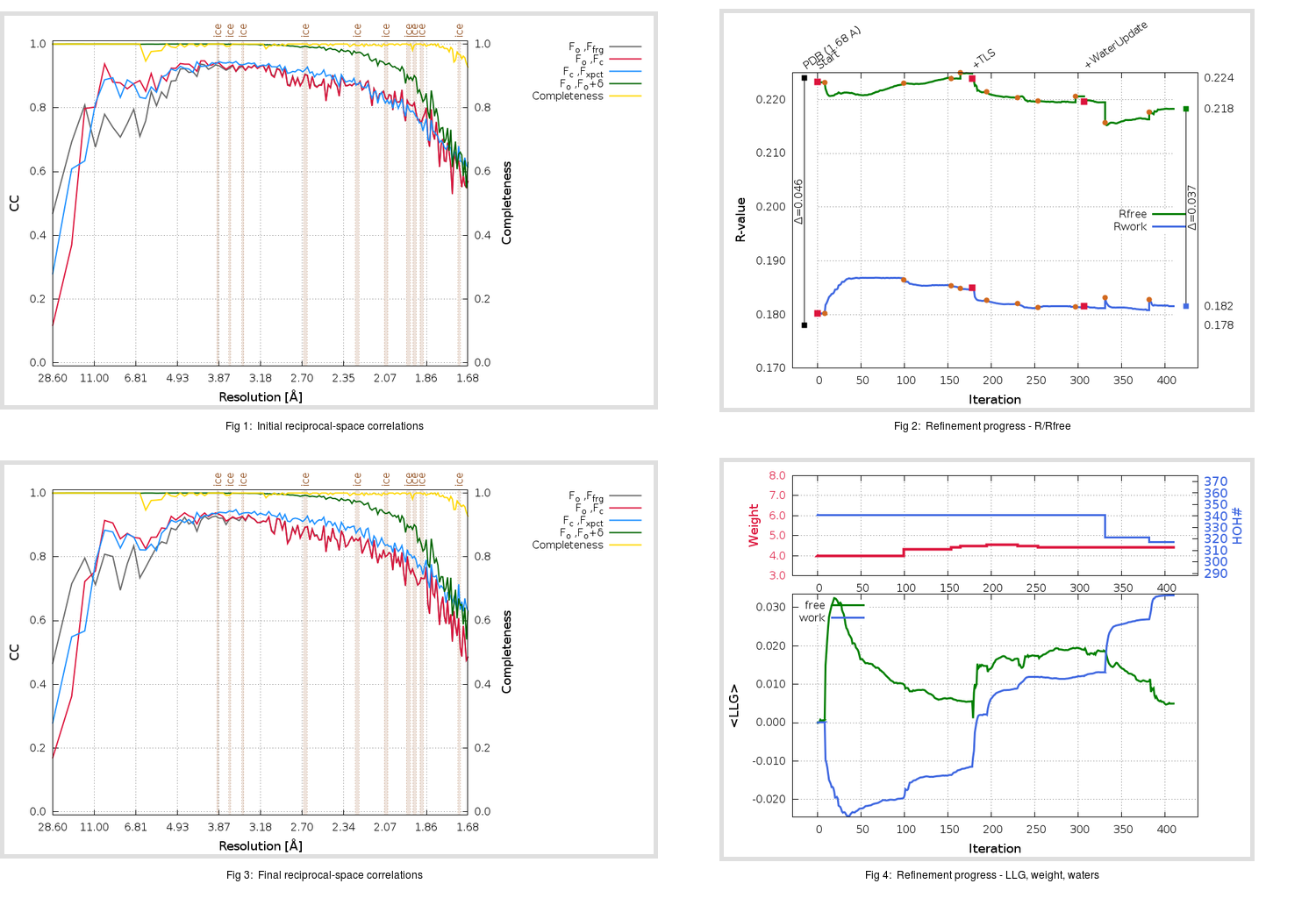Content:

## Deposited

` `
 Date deposited Date data collection Resolution R, Rfree 20200315 20200227 1.68 0.1760 0.2240

Molprobity (CCP4 7.0 version) summary:

```Ramachandran outliers =   0.33 %
favored =  98.68 %
Rotamer outliers      =   0.76 %
C-beta deviations     =     1
Clashscore            =   4.86
RMS(bonds)            =   0.0134
RMS(angles)           =   1.85
MolProbity score      =   1.25
Resolution            =   1.68
R-work                =   0.1760
R-free                =   0.2240
```

```Number of waters      =   341

<B> (all atoms) =   28.28 ( sd =   10.04 ) for       2736 non-hydrogen atoms
<B>   (protein) =   26.54 ( sd =    8.74 ) for       2370 non-hydrogen atoms
<B>     (water) =   39.35 ( sd =   10.78 ) for        341 non-hydrogen atoms
<B>    (others) =   41.94 ( sd =   10.72 ) for         25 non-hydrogen atoms

B min/max       (all non-hydrogen atoms) =   14.41 /   78.79
B min/max   (protein non-hydrogen atoms) =   14.41 /   74.98
B min/max     (water non-hydrogen atoms) =   15.51 /   78.79
B min/max     (other non-hydrogen atoms) =   26.38 /   51.64
```

## BUSTER (re-)refinement

` `

Molprobity (CCP4 7.0 version) summary:

```Ramachandran outliers =   0.33 %
favored =  98.68 %
Rotamer outliers      =   0.76 %
C-beta deviations     =     0
Clashscore            =   2.54
RMS(bonds)            =   0.0118
RMS(angles)           =   1.58
MolProbity score      =   1.04
Resolution            =   1.68
R-work                =   0.1816
R-free                =   0.2183
```

```Number of waters      =   317

<B> (all atoms) =   28.12 ( sd =    9.18 ) for       2712 non-hydrogen atoms
<B>   (protein) =   26.27 ( sd =    7.19 ) for       2370 non-hydrogen atoms
<B>     (water) =   40.51 ( sd =   11.48 ) for        317 non-hydrogen atoms
<B>    (others) =   45.61 ( sd =   12.21 ) for         25 non-hydrogen atoms

B min/max       (all non-hydrogen atoms) =   12.96 /   94.27
B min/max   (protein non-hydrogen atoms) =   12.96 /   56.44
B min/max     (water non-hydrogen atoms) =   14.36 /   94.27
B min/max     (other non-hydrogen atoms) =   28.50 /   54.67
```

Refinement progression:Results:

` `
 File Remark 5REB_aB_refine.01_03_refine.pdb.gz exact refinement commands are in header 5REB_aB_refine.01_03_refine.mtz.gz including original deposited data and several re-refinement map coefficients 5REB_aB_refine.01_03_BUSTER_model.cif.gz including any non-standard compound restraints 5REB_aB_refine.01_03_BUSTER_refln.cif.gz